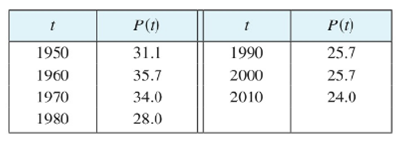Chapter 2, Problem 50RE### Single Variable Calculus: Early Tr...

8th Edition
James Stewart
ISBN: 9781305270343

#### Solutions

Chapter
Section### Single Variable Calculus: Early Tr...

8th Edition
James Stewart
ISBN: 9781305270343
Textbook Problem

# Let P(t) be the percentage of Americans under the age of 18 at time t. The table gives values of this function in census years from 1950 to 2010.(a) What is the meaning of P'(t)? What are its units?(b) Construct a table of estimated values for P'(t).(c) Graph P and P'(d) How would it be possible to get more accurate values for P'(t)?

(a)

To determine

To explain: The meaning of P(t) and write its unit.

Explanation

The term P(t) is the rate in change of percentage of a Americans whose age is below 18 years.

Numerically, P(t)=lim

(b)

To determine

To construct: The table of estimated values of P(t).

(c)

To determine

To sketch: The graph of P(t) and P(t).

(d)

To determine

To find: The method to get more accurate values of P(t).

### Still sussing out bartleby?

Check out a sample textbook solution.

See a sample solution

#### The Solution to Your Study Problems

Bartleby provides explanations to thousands of textbook problems written by our experts, many with advanced degrees!

Get Started

#### Subtract the following numbers. 21. $206 minus$58

Contemporary Mathematics for Business & Consumers

#### Proof prove that abx2dx=b3a33.

Calculus: Early Transcendental Functions (MindTap Course List)

#### For f(x) = x3 + 7x, df = _____. a) (x3 + 7x) dx b) (3x2 + 7) dx c) 3x2 + 7 d) x3 + 7x + dx

Study Guide for Stewart's Single Variable Calculus: Early Transcendentals, 8th